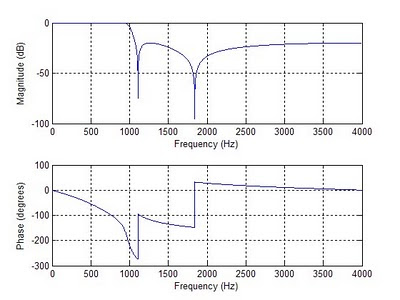## Friday, February 12, 2010

### DSP: Lowpass Elliptical Filtering

The following function creates a lowpass elliptic filter. By implementing this filter in a function we can easily call the lowpass filter in our code, passing our signal "x", sample frequency "fs", and desired cutoff frequency "fc".`function [y,N,B,A]= lowpass(x,fs,fc);% Description: This function uses an elliptical filter% to filter out high frequencies.%% [y,N,B,A] = lowpass(x,fs,fc)%% Variable Definition:% x: Input signal% fs: Input signal sampling frequency in Hz% fc: cutoff frequency%% y: Filtered signal (output)% N: Filter order% B: Feedforward (FIR) coefficients% A: Feedback (IIR) coefficientsRp = 0.5; % Ripple in dB (passband)Rs = 20; % Attenuationmf = fs/2; % Maximum frequencyWp = fc/mf; % Cuttoff frequencyWs = 1.2 * Wp; %Stopband frequency% First, determine the minimum filter order to meet specifics[N,Wn] = ellipord(Ws,Wp,Rp,Rs);% Next, Design the filter (find the coefficients)[B,A] = ellip(N,Rp,Rs,Wn,'low');% Now, apply the filter (filter the signal)y = filtfilt(B,A,x);% Plot the filterfigure('Color',[1 1 1]);freqz(B,A,2^14,fs);`